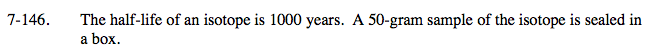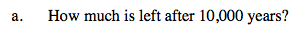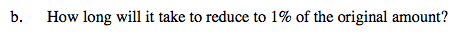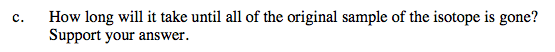### Home > A2C > Chapter 7 > Lesson 7.2.4 > Problem7-146

7-146.Half-life means time in which half of the original 50 grams will remain.

y = abn

a is the initial value,
b is the multiplier (0.5 since we are working with a half-life),
n is the number of thousands of years, and
y is the amount of the isotope that remains (in grams).

The equation is y = 50(0.5)x. Substitute 10 for x.

Use the equation below, where x is the number of years.

$y = 50(0.5)^{\frac{x}{1000}}$Use the equation from the help for part (a).
In this case, y = 1% of 50 and you are solving for x.

≈ 6600 years$0=50\left( \frac{1}{2}\right) ^x$